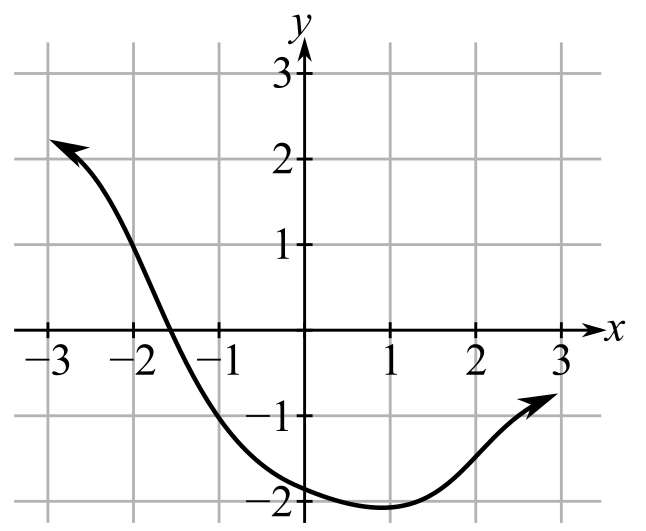Chapter 10.2, Problem 1EFinite Mathematics and Applied Cal...

7th Edition
Stefan Waner + 1 other
ISBN: 9781337274203

Solutions

Chapter
SectionFinite Mathematics and Applied Cal...

7th Edition
Stefan Waner + 1 other
ISBN: 9781337274203
Textbook Problem

In Exercises 1–14 the graph of a function f is given. Determine whether f is continuous on its domain. If it is not continuous on its domain, say why. [HINT: See Quick Examples 1–4.]To determine

Whether the function f is continuous on its domain and give reason if it is not continuous. The graph of function f is as follows:Explanation

Given Information:

The provided graph is:

Consider the provided graph:

Still sussing out bartleby?

Check out a sample textbook solution.

See a sample solution

The Solution to Your Study Problems

Bartleby provides explanations to thousands of textbook problems written by our experts, many with advanced degrees!

Get Started

What is the value of (X + 1) for the following scores: 0, 1, 4, 2? a. 8 b. 9 c. 11 d. 16

Essentials of Statistics for The Behavioral Sciences (MindTap Course List)

For , f′(x) =

Study Guide for Stewart's Multivariable Calculus, 8th

Sometimes, Always, or Never: If f(x) is continuous on [a, b], then abf(x) dx exists.

Study Guide for Stewart's Single Variable Calculus: Early Transcendentals, 8th